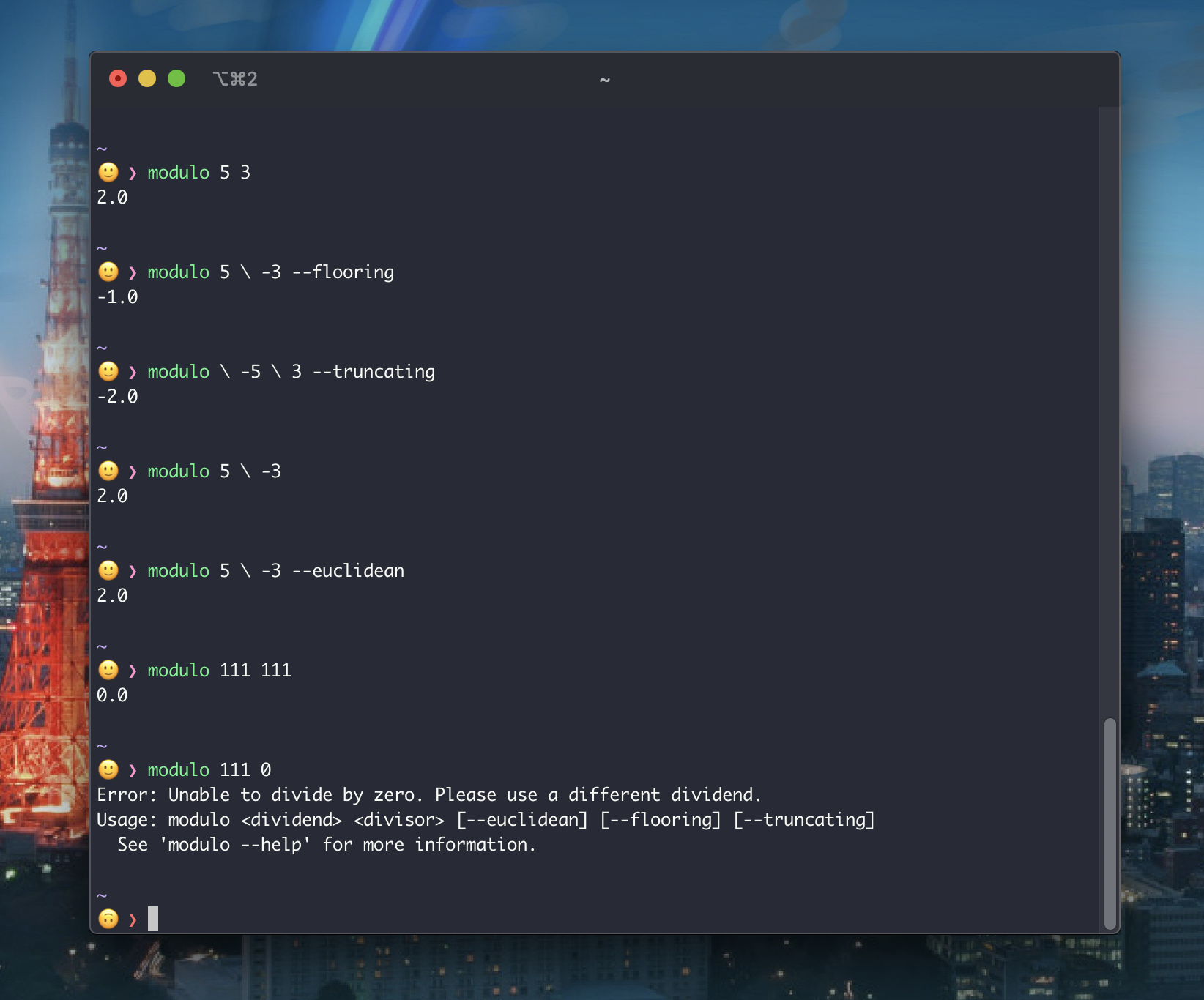## ModulusOperandi

Declarative, multi-algorithm modular arithmetic for Swift Integers and Floating-Point types.

Modular arithmetic algorithms come in variants that use either Euclidean, truncating, or flooring division. Furthermore, Swift's built-in % operator — while used as a modulus in some languages — is strictly used as a remainder operator.

These nuances can lead modular arithmetic code that's ambiguous in both intent and correctness — which is what ModulusOperandi attempts to solve.

## Features

• ✅ Declarative API that allows for choosing between Euclidean, Truncating, or Flooring Modular Arithmetic.
• ✅ Euclidean by default.
• ✅ Support for conformances to `BinaryInteger` and `FloatingPointInteger`.
• ✅ Command Line tool for performing calculations in the Terminal.

## Installation

### Xcode Projects

Select `File` -> `Swift Packages` -> `Add Package Dependency` and enter `https://github.com/CypherPoet/ModulusOperandi`.

### Swift Package Manager Projects

You can add `ModulusOperandi` as a dependency in your `Package.swift` file:

``````let package = Package(
//...
dependencies: [
.package(url: "https://github.com/CypherPoet/ModulusOperandi", from: "0.2.2"),
],
//...
)
``````

Then simply `import ModulusOperandi` wherever you’d like to use it.

## Usage

After importing `ModulusOperandi` in a file, types that conform to `BinaryInteger` and `FloatingPointInteger` will be extended with a `modulus` function.

This function treats its value as the `dividend` and takes a `divisor` of the same type. It also takes an optional `mode` argument to choose between Euclidean, Truncating, or Flooring Modular Arithmetic.

By default, the `mode` will be Euclidean

``````import ModulusOperandi

let dividend = 5
let divisor = 3

dividend.modulo(divisor)   // 2
dividend.modulo(-divisor)  // 2
-dividend.modulo(divisor)  // -2
-dividend.modulo(-divisor) // -2

// Same as...
dividend.modulo(divisor, mode: .euclidean)     // 2
dividend.modulo(-divisor, mode: .euclidean)    // 2
-dividend.modulo(divisor, mode: .euclidean)    // -2
-dividend.modulo(-divisor, mode: .euclidean)   // -2
``````
``````import ModulusOperandi

let dividend = 5
let divisor = 3

dividend.modulo(3, mode: .flooring)    // 2
dividend.modulo(-3, mode: .flooring)   // -1
-dividend.modulo(3, mode: .flooring)   // -2
-dividend.modulo(-3, mode: .flooring)  // 1
``````
``````import ModulusOperandi

let dividend = 5
let divisor = 3

dividend.modulo(3, mode: .truncating)    // 2
dividend.modulo(-3, mode: .truncating)   // 2
-dividend.modulo(3, mode: .truncating)   // -2
-dividend.modulo(-3, mode: .truncating)  // -2
``````

## Command Line Tool`ModulusOperandi` also ships with a command line tool that lets you perform calculations directly from the command line.

To install it, clone the project and run `make`:

``````\$ git clone [email protected]:CypherPoet/ModulusOperandi.git
\$ cd ModulusOperandiCLI
\$ make
``````

The command line tool will be installed as `modulo`, and running `modulo --help` will present some helpful usage instructions:

``````modulo --help
``````
``````OVERVIEW: Multi-algorithm modular arithmetic for Swift integers and
floating-Point types.

Modular arithmetic algorithms come in variants that use either Euclidean,
truncating, or flooring division.

This tool acts as a CLI for the `ModulusOperandi` Swift package -- which allows
you to perform modular arithmetic according to your desired algorithm.

📝 Note on Negative Numbers
----------------------------------------------

To use negative numbers, prefix the argument with `\ ` (including the space).

For example, -5 mod 4 would be passed as:
modulo \ -5 4

-5 mod -4 would be passed as:
modulo \ -5 \ -4

----------------------------------------------
- https://en.wikipedia.org/wiki/Modulo_operation#Variants_of_the_definition

USAGE: modulo  [--euclidean] [--flooring] [--truncating]

ARGUMENTS:
The dividend to perform division against.
The divisor to use as a "modulus".

OPTIONS:
--euclidean/--flooring/--truncating
The algorithm to use for computing results. (default:
euclidean)
--version               Show the version.
-h, --help              Show help information.

``````

### Negative Numbers

Disambiguating negative numbers from argument flags is a notorious challenge for Command Line interfaces. Currently, support for this in Swift's Argument Parser appears to be an ongoing area of development, In the meantime, though, the `modulo` command can take negative-number arguments via some clever escape syntax.

Simply prefix any negative number with `\` (including the space). Like so:

`-5 mod 4`:

``````modulo \ -5 4
``````

`-5 mod -4`:

``````modulo \ -5 \ -4
``````

`5 mod -4`:

``````modulo 5 \ -4
``````